## Alternative Form for the Frenet Equations

Theorem

The Frenet equations can be written in the form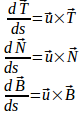Proof

For a curve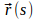the Frenet equations are

1.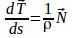where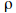is a scalar (called the radius of curvature) and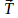is the unit tangent to the curve.

2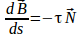where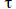is a scalar called the torsion and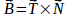3.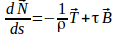where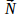is a unit normal to the curve.

Equating the expressions for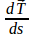gives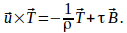Substituteto give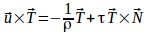The vectors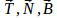form a right handed coordinate system so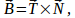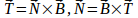and we can write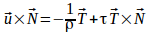as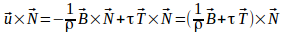hence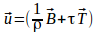Hence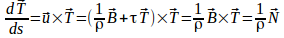(Eq 1. above) and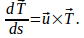Subsituteinto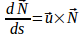to obtain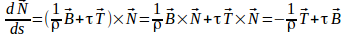which is equation 3.

Subsituteinto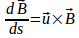to obtain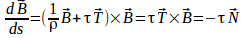which is Eq 2. above.